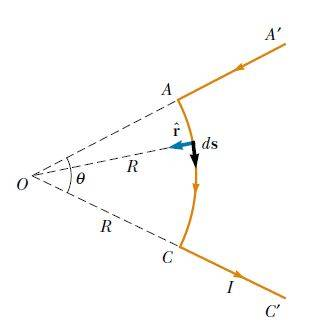# Biot–Savart Law Problem

22990atinesh

## Homework Statement

Calculate the magnetic field at point O for the current-carrying wire segment shown in Figure. The wire consists of two straight portions and a circular arc of radius R, which subtends an angle Ɵ. The arrowheads on the wire indicate the direction of the current(Biot–Savart Law).2. The attempt at a solution

##dB = \frac {μ I}{4π} \frac {ds}{R^2}## - I

##B = \frac {μ I}{4π R^2} \int ds = \frac {μ I}{4π R^2} s = \frac {μ I}{4π R} Θ##

Since s=RΘ

I'm little bit confused in the calcualtion of ds in equation I.
##|\vec {ds} \times \hat r| = |\vec {ds}|.1.sin 90^o = ds##
Does ds here represent the magnitude of the vector perpendicular to the vector ##\vec {ds}## and ##\hat r## or it represents the magnitude of orginal vector ##\vec {ds}##.

## Answers and Replies

dauto
ds is the magnitude of the vector ds what else could it be?

22990atinesh
ds is the magnitude of the vector ds what else could it be?

I know ds is magnitude of ##\vec{ds}##. My doubt is What does it represents. Does ds here represent the magnitude of the vector perpendicular to the vector ##\vec{ds}## and ##\hat{r}## or it represents the magnitude of orginal vector ##\vec{ds}##.

Gold Member
ds is the magnitude of the original vector ##\vec{ds}## and the direction of ##\vec {ds} \times \hat r## is the direction of ##d\vec{B}## due to the elemental current carrying wire.

22990atinesh
ds is the magnitude of the original vector ##\vec{ds}## and the direction of ##\vec {ds} \times \hat r## is the direction of ##d\vec{B}## due to the elemental current carrying wire.

Thanx I get it